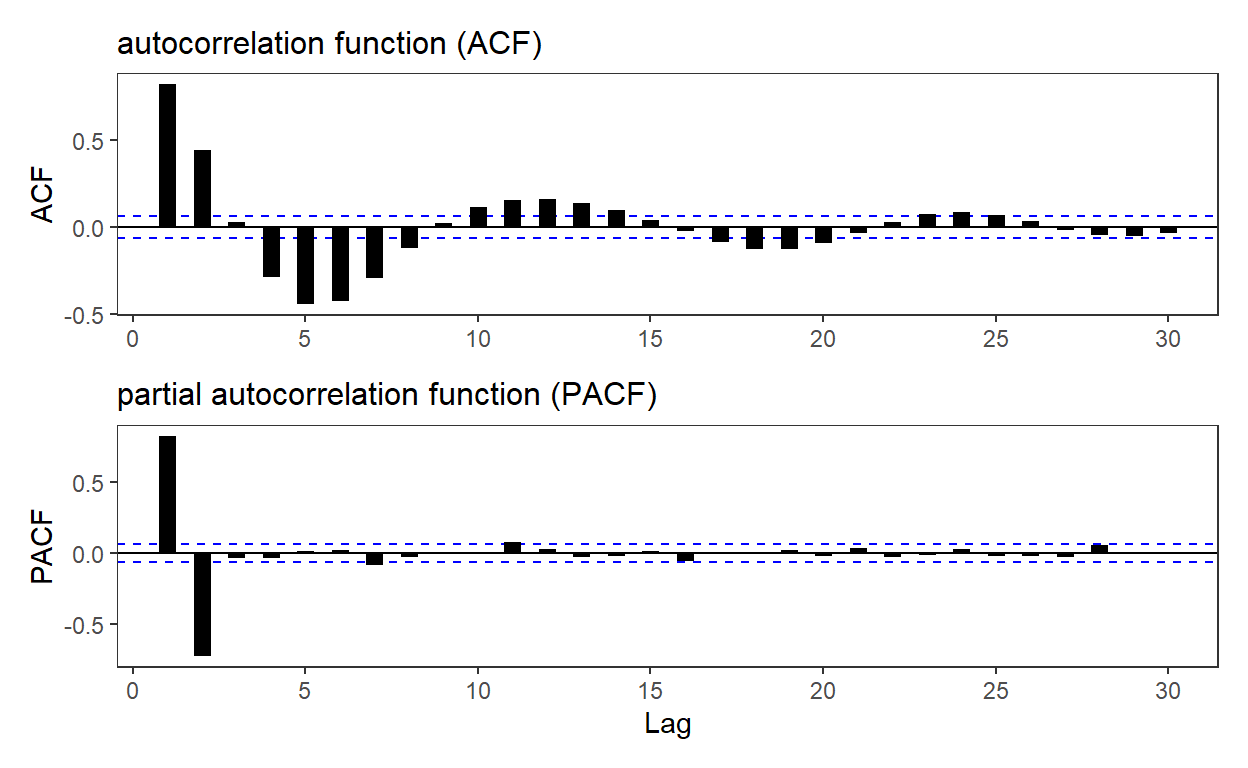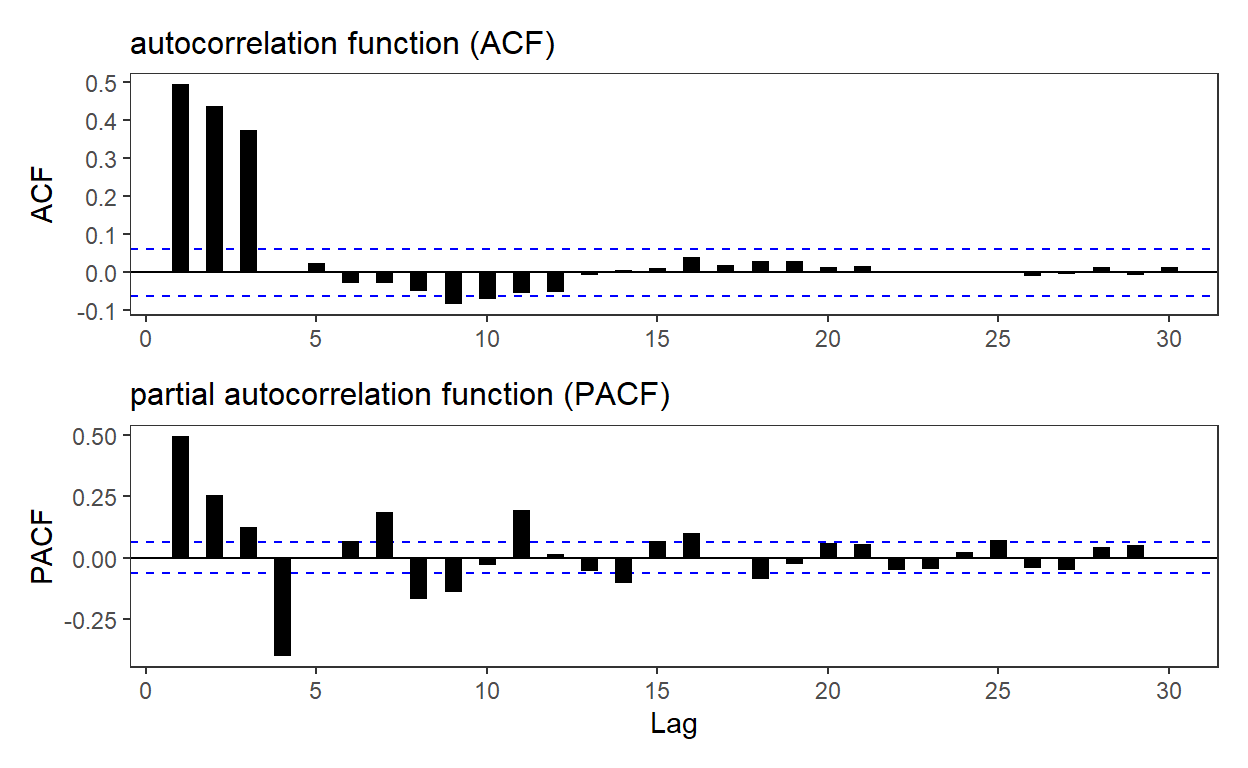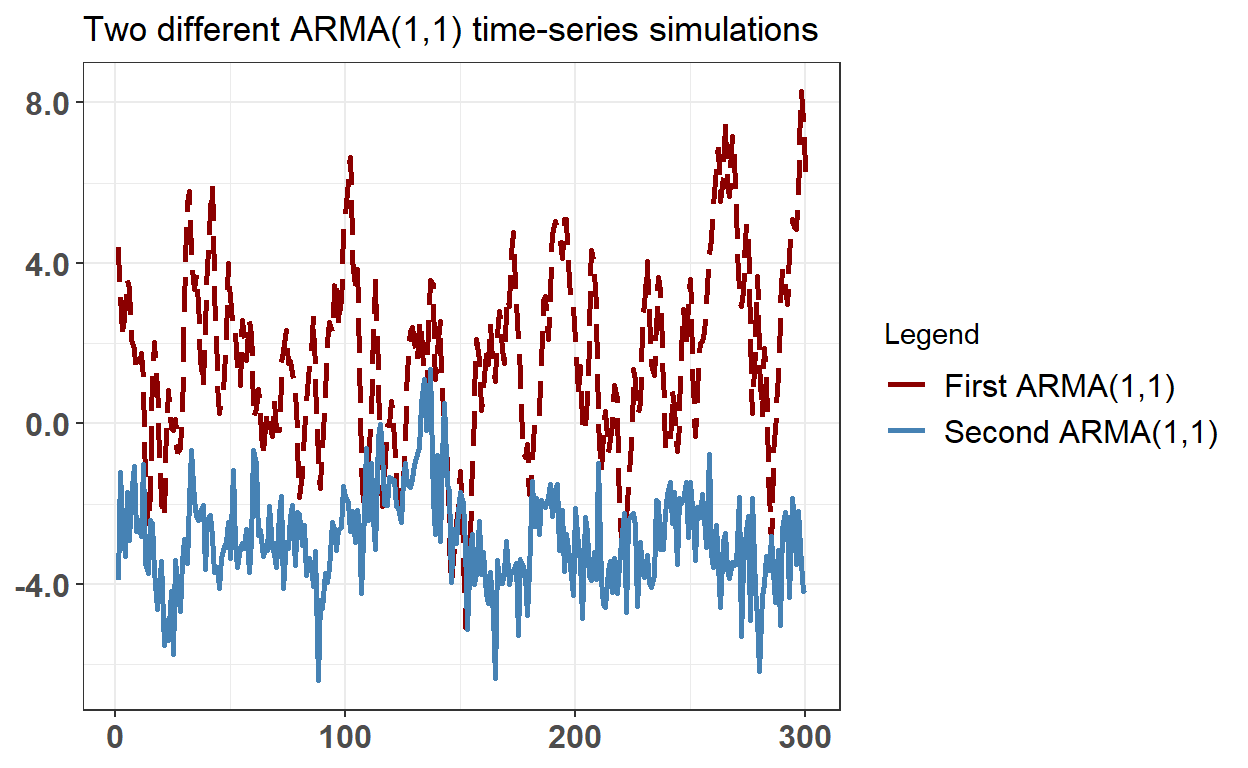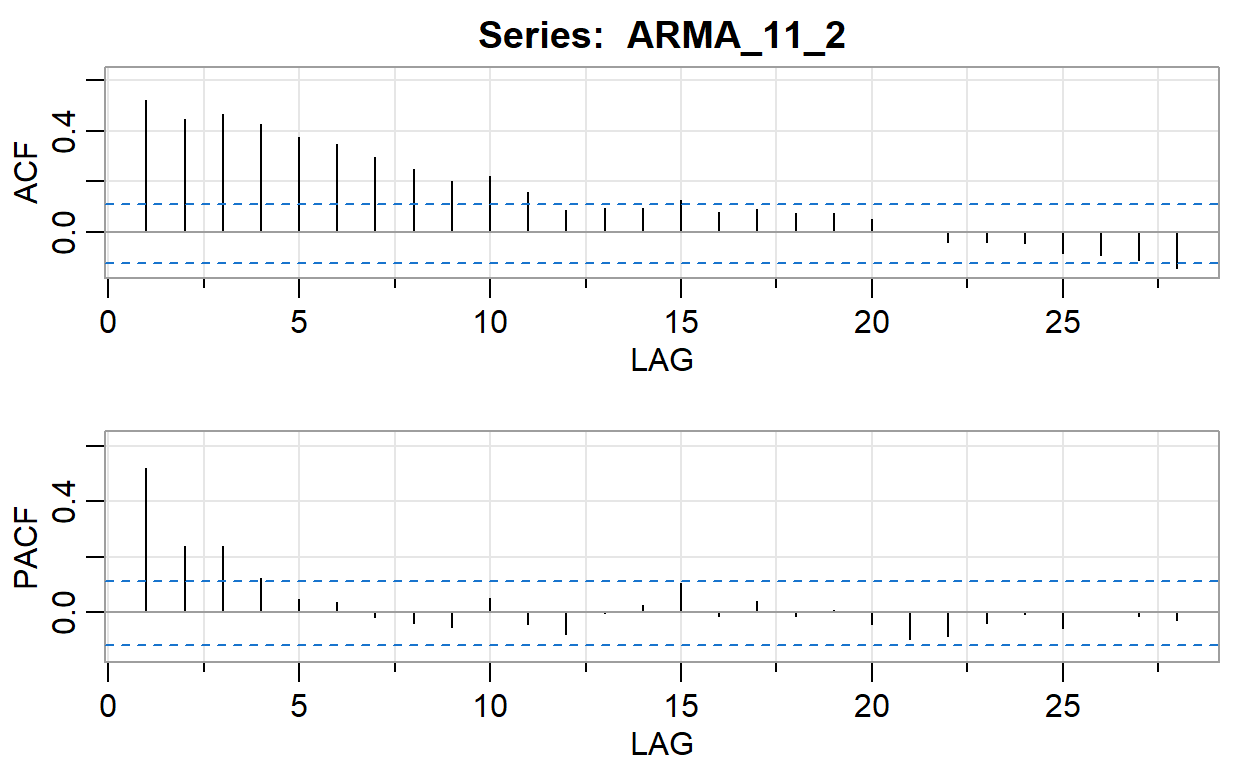# BT PQ P1.T2.20.23 (SET) autoregressive moving average (ARMA) models

ARMA(p,q) combines an AR(p) and MA(q)

David Harper https://www.bionicturtle.com/
2022-01-16

Learning objectives

• Explain mean reversion and calculate a mean-reverting level.
• Define and describe the properties of autoregressive moving average (ARMA) processes.
• Describe the application of AR, MA and ARMA processes.

``````library(tidyverse)
library(forecast) # incl ggACF()
# library(cowplot)
library(patchwork)
``````

20.23.1. Below are plotted the autocorrelation function (ACF) and partial autocorrelation function (PACF) for a simulated time-series process. Please note that the horizontal dashed blue lines represent the 95.0% confidence interval … Which of the following time-series models is most likely described by these ACF and PACF plots?

``````set.seed(66)

AR_param_1 = 1.4
AR_param_2 = -0.7
AR_intercept = 0
AR_n <- 1000

theme_set(theme_bw())

# arima.sim model c(p, d, q)
# p = AR order
# d = difference
# q = MA order
# Generate an AR(2) with parameters, AR_param_1 and AR_param_2
AR <- arima.sim(model=list(order=c(2,0,0), ar = c(AR_param_1, AR_param_2)), n = AR_n, mean = AR_intercept)

p3 <- ggAcf(AR) +
geom_segment(size = 3) +
labs(
title = "autocorrelation function (ACF)"
) + theme(
plot.title = element_text(size = 12),
axis.title.x = element_blank(),
panel.grid = element_blank()
)
p4 <- ggPacf(AR) +
geom_segment(size = 3) +
labs(
title = "partial autocorrelation function (PACF)"
) + theme(
plot.title = element_text(size = 12),
panel.grid = element_blank()
)

# Please note plot is shrunk to 86% on copy/paste
p3 / p4
``````20.23.2. Below are plotted the autocorrelation function (ACF) and partial autocorrelation function (PACF) for a simulated time-series process. Please note that the horizontal dashed blue lines represent the 95.0% confidence interval … Which of the following time-series models is most likely described by these ACF and PACF plots?

``````set.seed(19)

MA_n = 1000
MA_mean = 0
MA_weight_1 = 0.4
MA_weight_2 = 0.6
MA_weight_3 = 0.8

# # Generate an MA(3) with weights MA_weight_1, MA_weight_2, and MA_weight_3
MA <- arima.sim(model=list(order = c(0,0,3), ma = c(MA_weight_1, MA_weight_2, MA_weight_3)), n = MA_n, mean = MA_mean)

p5 <- ggAcf(MA) +
geom_segment(size = 3) +
# geom_hline(yintercept = c(ci2, -ci2), color = "darkblue", linetype = "dashed", size = 2 ) +
labs(
title = "autocorrelation function (ACF)"
) + theme(
plot.title = element_text(size = 12),
axis.title.x = element_blank(),
panel.grid = element_blank()
)
p6 <- ggPacf(MA) +
geom_segment(size = 3) +
labs(
title = "partial autocorrelation function (PACF)"
) + theme(
plot.title = element_text(size = 12),
panel.grid = element_blank()
)

# Please note plot is shrunk to 86% on copy/paste
p5 / p6
``````20.23.3. An ARMA(1,1) process evolves according to Y(t) = ẟ + ϕ*Y(t-1) + θ*ε(t-1) + ε(t). The plot below contains two 300-step ARMA(1,1) processes that differ only in their coefficients …

Which of the following statements is TRUE about the two ARMA(1,1) simulations above?

1. Both of the ARMA(1,1) processes are likely to exhibit slow decay in both the ACF and PACF
2. Both of the ARMA(1,1) processes are likely to cut off sharply at lag 1 in both the ACF and PACF
3. The first ARMA(1,1) process (i.e., plotted with a red dashed line) cannot be covariance-stationary
4. The unconditional mean of both ARMA(1,1) processes must be zero because all ARMA(1,1) have a long-run mean of zero
``````library(astsa)
set.seed(9)

ar_11_1 = 0.8
ma_11_1 = 0.6
arma_11_1_mu = 2
sim_n = 300

ar_11_2 = 0.9
ma_11_2 = -0.5
arma_11_2_mu = -3

# ar_22 = c(1.4, -0.6)
# ma_22 = c(0.9, 0.6)

ARMA_11_1 <- arima.sim(model=list(order = c(1, 0, 1), ar = ar_11_1, ma = ma_11_1), n = sim_n) + arma_11_1_mu
ARMA_11_2 <- arima.sim(model=list(order = c(1, 0, 1), ar = ar_11_2, ma = ma_11_2), n = sim_n) + arma_11_2_mu
# ARMA_22 <- arima.sim(model=list(order = c(2, 0, 2), ar = ar_22, ma = ma_22), n = 200)

# ts.plot(ARMA_11, ARMA_22)

time_arma <- bind_cols(ARMA_11_1, ARMA_11_2) %>% rowid_to_column() %>%
rename(y_ARMA_11_1 = ...1, y_ARMA_11_2 = ...2)

theme_set(theme_bw())

colors <- c("First ARMA(1,1)" = "darkred", "Second ARMA(1,1)" = "steelblue")

time_arma %>% ggplot(aes(x = rowid)) +
geom_line(aes(y = y_ARMA_11_1, color = "First ARMA(1,1)"), size = 1, linetype = "longdash") +
geom_line(aes(y = y_ARMA_11_2, color = "Second ARMA(1,1)"), size = 1) +
scale_y_continuous(labels = scales::number_format(accuracy = 0.1)) +
theme(
axis.title.y = element_blank(),
axis.title.x = element_blank(),
axis.text.x = element_text(size = 12, face = "bold"),
axis.text.y = element_text(size = 12, face = "bold"),
legend.position = "right",
legend.text = element_text(size = 12)
) + labs(
title = "Two different ARMA(1,1) time-series simulations",
color = "Legend"
) +
scale_color_manual(values = colors)
````````````arma_11_1_mulr = arma_11_1_mu/(1 - ar_11_1)
arma_11_2_mulr = arma_11_2_mu/(1 - ar_11_2)

# mu_arma_22 = 10
#ARMA_22 <- arima.sim(model=list(order = c(2, 0, 2), ar = c(0.4, 0.2), ma = c(0.5,0.6)), n = 200, mean = mu_arma_22)
# ts.plot(ARMA_22)
# acf2(ARMA_22)
# mean_arma_22 = mu_arma_22/(1 - 0.4 - 0.2)

acf2(ARMA_11_1)
````````````     [,1]  [,2] [,3]  [,4] [,5] [,6]  [,7]  [,8]  [,9] [,10] [,11]
ACF  0.86  0.65 0.50  0.40 0.33 0.29  0.24  0.18  0.11  0.03 -0.05
PACF 0.86 -0.39 0.28 -0.11 0.07 0.05 -0.07 -0.06 -0.01 -0.13 -0.06
[,12] [,13] [,14] [,15] [,16] [,17] [,18] [,19] [,20] [,21]
ACF  -0.12 -0.16 -0.17 -0.17 -0.15 -0.12 -0.10 -0.11 -0.13 -0.15
PACF -0.01 -0.03  0.01  0.03  0.06  0.00  0.01 -0.08 -0.03 -0.05
[,22] [,23] [,24] [,25] [,26] [,27] [,28]
ACF  -0.14 -0.08 -0.02  0.03  0.05  0.07  0.11
PACF  0.10  0.06  0.00  0.04 -0.05  0.13  0.07``````
``````acf2(ARMA_11_2)
````````````     [,1] [,2] [,3] [,4] [,5] [,6]  [,7]  [,8]  [,9] [,10] [,11]
ACF  0.52 0.45 0.46 0.43 0.37 0.35  0.30  0.25  0.20  0.22  0.16
PACF 0.52 0.24 0.24 0.12 0.05 0.04 -0.02 -0.04 -0.05  0.05 -0.04
[,12] [,13] [,14] [,15] [,16] [,17] [,18] [,19] [,20] [,21]
ACF   0.09  0.09  0.10  0.13  0.08  0.09  0.08  0.07  0.05  0.00
PACF -0.08  0.00  0.03  0.10 -0.01  0.04 -0.01  0.01 -0.04 -0.09
[,22] [,23] [,24] [,25] [,26] [,27] [,28]
ACF  -0.04 -0.04 -0.04 -0.08 -0.09 -0.11 -0.14
PACF -0.09 -0.04 -0.01 -0.06  0.00 -0.01 -0.03``````